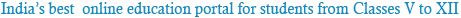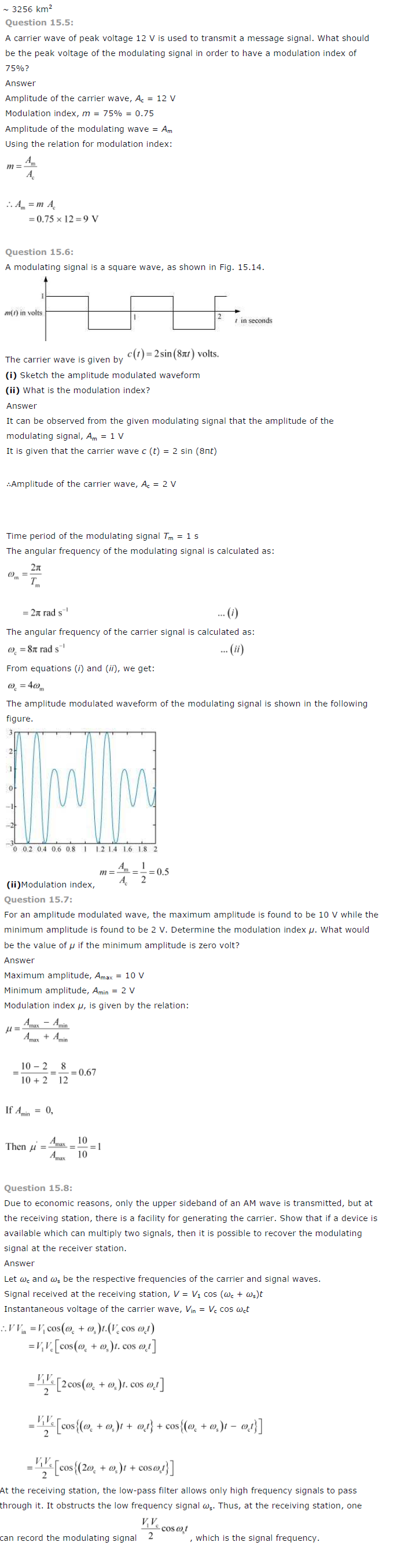### NCERT Solutions for Class 12th Physics Chapter 15: Communication Systems

Question 15.1:
Which of the following frequencies will be suitable for beyond-the-horizon communication
using sky waves?
(a) 10 kHz
(b) 10 MHz
(c) 1 GHz
(d) 1000 GHz
10 MHz
For beyond-the-horizon communication, it is necessary for the signal waves to travel a large distance. 10 KHz signals cannot be radiated efficiently because of the antenna size.
The high energy signal waves (1GHz − 1000 GHz) penetrate the ionosphere. 10 MHz frequencies get reflected easily from the ionosphere. Hence, signal waves of such frequencies are suitable for beyond-the-horizon communication.

Question 15.2:
Frequencies in the UHF range normally propagate by means of:
(a) Ground waves.
(b) Sky waves.
(c) Surface waves.
(d) Space waves.
Space waves
Owing to its high frequency, an ultra high frequency (UHF) wave can neither travel along the trajectory of the ground nor can it get reflected by the ionosphere. The signals having UHF are propagated through line-of-sight communication, which is nothing but space wave propagation.

Question 15.3:
Digital signals
(i) Do not provide a continuous set of values,
(ii) Represent values as discrete steps,
(iii) Can utilize binary system, and
(iv) Can utilize decimal as well as binary systems.
Which of the above statements are true?
(a) (i) and (ii) only
(b) (ii) and (iii) only
(c) (i), (ii) and (iii) but not (iv)
(d) All of (i), (ii), (iii) and (iv).
A digital signal uses the binary (0 and 1) system for transferring message signals. Such a system cannot utilise the decimal system (which corresponds to analogue signals).
Digital signals represent discontinuous values.

Question 15.4:
Is it necessary for a transmitting antenna to be at the same height as that of the receiving antenna for line-of-sight communication? A TV transmitting antenna is 81m tall. How much service area can it cover if the receiving antenna is at the ground level?
Line-of-sight communication means that there is no physical obstruction between the transmitter and the receiver. In such communications it is not necessary for the transmitting and receiving antennas to be at the same height.
Height of the given antenna, h = 81 m
Radius of earth, R = 6.4 × 10^6 m
For range, d = 2Rh, the service area of the antenna is given by the relation:
A = πd2
= π (2Rh)
= 3.14 × 2 × 6.4 × 10^6 × 81
= 3255.55 × 10^6 m^2
= 3255.55Powered by: iVerticals Web Technology Pvt Ltd